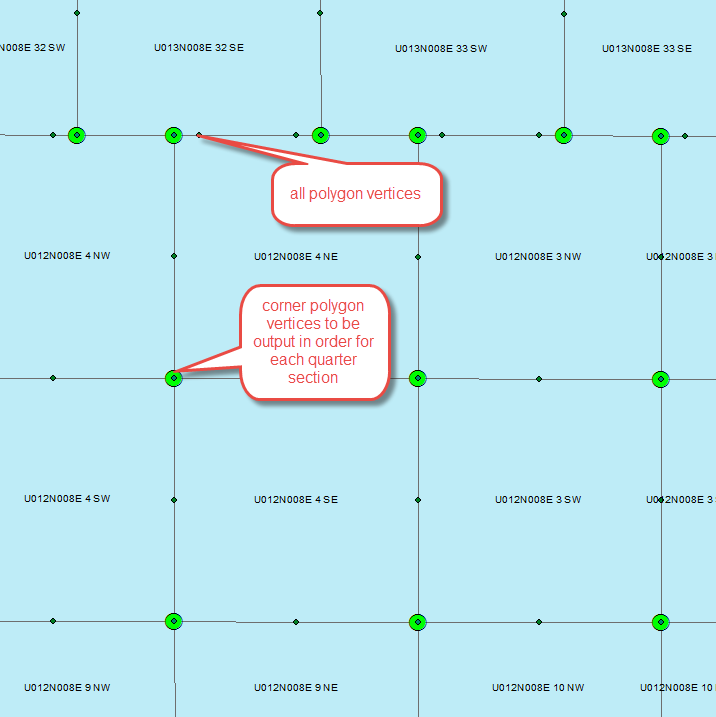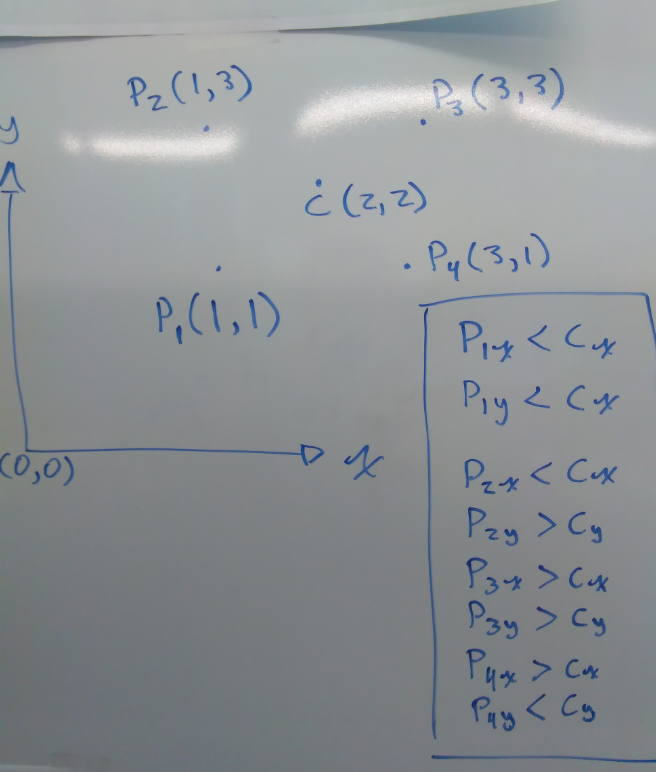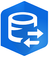# How to order point of polygon clockwise

13125
8
10-12-2016 10:51 AMbyMVP Regular Contributor

Is there a fast way to order points clockwise? I only want the corner vertices. I would like the first point to be in the SW corner of the quarter section or at least in a consistent location....1 Solution

Accepted SolutionsbyMVP Regular Contributor

Thanks everyone for the great feedback!

So, I went with this solution in the end. Only works for my quarter section special case. But maybe someone will find it useful.

``````import arcpy

points = r"xxxx"
center = r"xxxx"
csv_path = r"xxxx"
dec_field = "DESCRIPTION"

csv = open(csv_path, "w")

search = arcpy.SearchCursor(points)
points_list = []
for row in search:
points_list.append((row.getValue(dec_field), row.getValue("POINT_X"), row.getValue("POINT_Y")))
del search

search = arcpy.SearchCursor(center)
center_list = []
for row in search:
center_list.append((row.getValue(dec_field), row.getValue("POINT_X"), row.getValue("POINT_Y")))
del search

k = 0
order_list = []
order_center = None
for dxy_p in points_list:
k += 1
order_list.append(dxy_p)
if k == 4:
# find center point
for dxy_c in center_list:
if dxy_c == order_list:
order_center = dxy_c
break
for dxy_o in order_list:
if dxy_o < order_center and dxy_o < order_center:
csv.write(dxy_o + ", " + str(dxy_o) + ", " + str(dxy_o) + ", " + "1\n")
if dxy_o < order_center and dxy_o > order_center:
csv.write(dxy_o + ", " + str(dxy_o) + ", " + str(dxy_o) + ", " + "2\n")
if dxy_o > order_center and dxy_o > order_center:
csv.write(dxy_o + ", " + str(dxy_o) + ", " + str(dxy_o) + ", " + "3\n")
if dxy_o > order_center and dxy_o < order_center:
csv.write(dxy_o + ", " + str(dxy_o) + ", " + str(dxy_o) + ", " + "4\n")
k = 0
order_list = []
dxy_c = None

csv.close()‍‍‍‍‍‍‍‍‍‍‍‍‍‍‍‍‍‍‍‍‍‍‍‍‍‍‍‍‍‍‍‍‍‍‍‍‍‍‍‍‍‍‍‍‍‍‍``````
8 RepliesbyMVP Esteemed Contributor

fast? hmmm  Well I suspect that this is a 'nice' versus a 'need' question, but the best way I have found to retain a consistent order is to sort a polygon's point list around the center of that list.

The method I have used is

• determine the center of the polygon (assuming convex polygons as in your case)
• calculate the direction angle to the points in the list
• sort that list by the angle (angles will go from -180 to + 180)
• flip the list so that they are sorted in reverse (+180 to -180) or sort in reverse in the first place.

All points will be sorted in clockwise order with the first point in the North West quadrant.  In your case since you only have 4 points and they are the corners, simply insert the last point (SW) into the first position in the listbyMVP Regular Contributor

Thanks for the feedback.

No, it is a need. Because it is going to a spatial application that is not so smart. I think your idea would work well.

I am thinking one could also do something like this (with the logic tests in the box):But I think I will have to script the solution. Sometimes I run to python as a first solution when there is a workflow that is out of the box that will do the same thing only faster...byMVP Honored Contributor

If you have standard or advanced licensing, I believe you could use Simplify Polygon to get only the corner points, add XY coordinates for each point, then use a tool like Summary Statistics to get specific corners (SW would MIN Y, MIN X, grouped by polygon ID).

edit: I wrote that last part too fast. The SW point would be the nearest of the four points to MIN X, MIN Y, not MIN X, MIN Y. You could also find the corner directions by assigning each corner an angle from the centroid and grouping that way.byEsri Regular Contributor

ArcGIS Data Interoperability extension has a GeometryValidator transformer that can enforce right (or left) hand rule orientation of polygon boundaries.byMVP Esteemed Contributor

If you plan on building polygons within ArcGIS from these ordered points, just be aware that the software will impose its own ordering scheme regardless of the ordering scheme you use when working with the vertices outside of the software.

``````>>> pg_ccw = arcpy.FromWKT('POLYGON((0 0, 10 0, 10 10, 0 10, 0 0))')
>>> pg_ccw.area
100.0
>>> pg_cw = arcpy.FromWKT('POLYGON((0 0, 0 10, 10 10, 10 0, 0 0))')
>>> pg_cw.area
100.0
>>> pg_ccw.WKT
u'MULTIPOLYGON (((0 0, 10 0, 10 10, 0 10, 0 0)))'
>>> pg_cw.WKT
u'MULTIPOLYGON (((0 0, 10 0, 10 10, 0 10, 0 0)))'  #Clockwise ordering is swapped to counter clockwise
>>> ‍‍‍‍‍‍‍‍‍‍‍``````byMVP Regular Contributor

Good to know thanks Joshua. The end product for me is a table.byMVP Esteemed Contributor

Send your polygons out to a numpy array... just the coordinates for testing.

Since your polygons will be properly ordered clockwise if you made them in ArcMap, then the process is a bit fiddly.

Consider a square defined by the coordinates in array 'a'.

I made 3 other polygons, rotated about the center so that the lower left corner of 'a' was in a different position.

When you have this inside NumPy, it is a matter of reordering the structured array so that the minimum of a 'sort' is used to 'roll' the points into their desired position.  This only works if the minimum is the lower left corner.  The sad thing that comparing and/or locating the minimum x value does not mean that you will have the lower left corner... consider a polygon leaning to the left at the top or the bottom left kicked in a bit.

Anyway, for some fun...  For the nice square.

``````    >>> a
array([(0, 0), (0, 1), (1, 1), (1, 0)],
dtype=[('x', '<i8'), ('y', '<i8')])
>>> b
array([(1, 0), (0, 0), (0, 1), (1, 1)],
dtype=[('x', '<i8'), ('y', '<i8')])
>>> c
array([(1, 1), (1, 0), (0, 0), (0, 1)],
dtype=[('x', '<i8'), ('y', '<i8')])
>>> d
array([(0, 1), (1, 1), (1, 0), (0, 0)],
dtype=[('x', '<i8'), ('y', '<i8')])
>>> a_i = a.argsort()
>>> b_i = b.argsort()
>>> c_i = c.argsort()
>>> d_i = d.argsort()
>>> a_i, b_i, c_i, d_i
(0, 1, 2, 3)
>>>
>>> # time to roll
>>> a
array([(0, 0), (0, 1), (1, 1), (1, 0)],
dtype=[('x', '<i8'), ('y', '<i8')])
>>> np.roll(b,-1)
array([(0, 0), (0, 1), (1, 1), (1, 0)],
dtype=[('x', '<i8'), ('y', '<i8')])
>>> np.roll(c, -2)
array([(0, 0), (0, 1), (1, 1), (1, 0)],
dtype=[('x', '<i8'), ('y', '<i8')])
>>> np.roll(d, -3)
array([(0, 0), (0, 1), (1, 1), (1, 0)],
dtype=[('x', '<i8'), ('y', '<i8')])‍‍‍‍‍‍‍‍‍‍‍‍‍‍‍‍‍‍‍‍‍‍‍‍‍‍‍‍‍‍‍‍``````

Now as a test, let's check the case where the bottom left isn't the minimum X and Y.

``````>>> a
array([(0.1, 0.1), (0.0, 1.0), (1.0, 1.0), (1.0, 0.0)],
dtype=[('x', '<f8'), ('y', '<f8')])
>>> b
array([(1.0, 0.0), (0.1, 0.1), (0.0, 1.0), (1.0, 1.0)],
dtype=[('x', '<f8'), ('y', '<f8')])
>>> c
array([(1.0, 1.0), (1.0, 0.0), (0.1, 0.1), (0.0, 1.0)],
dtype=[('x', '<f8'), ('y', '<f8')])
>>> d
array([(0.0, 1.0), (1.0, 1.0), (1.0, 0.0), (0.1, 0.1)],
dtype=[('x', '<f8'), ('y', '<f8')])
>>> a_i = a.argsort()
>>> b_i = b.argsort()
>>> c_i = c.argsort()
>>> d_i = d.argsort()
>>> a_i, b_i, c_i, d_i
(1, 2, 3, 0)
>>> a
array([(0.1, 0.1), (0.0, 1.0), (1.0, 1.0), (1.0, 0.0)],
dtype=[('x', '<f8'), ('y', '<f8')])
>>> np.roll(b, -1)
array([(0.1, 0.1), (0.0, 1.0), (1.0, 1.0), (1.0, 0.0)],
dtype=[('x', '<f8'), ('y', '<f8')])
>>> np.roll(c, -2)
array([(0.1, 0.1), (0.0, 1.0), (1.0, 1.0), (1.0, 0.0)],
dtype=[('x', '<f8'), ('y', '<f8')])
>>> np.roll(c, -3)
array([(0.0, 1.0), (1.0, 1.0), (1.0, 0.0), (0.1, 0.1)],
dtype=[('x', '<f8'), ('y', '<f8')])
>>> np.roll(d, -3)
array([(0.1, 0.1), (0.0, 1.0), (1.0, 1.0), (1.0, 0.0)],
dtype=[('x', '<f8'), ('y', '<f8')])‍‍‍‍‍‍‍‍‍‍‍‍‍‍‍‍‍‍‍‍‍‍‍‍‍‍‍‍‍‍‍‍‍``````

Looks ok-ish... but I would experiment with your 'rolling' just to make sure.byMVP Regular Contributor

Thanks everyone for the great feedback!

So, I went with this solution in the end. Only works for my quarter section special case. But maybe someone will find it useful.

``````import arcpy

points = r"xxxx"
center = r"xxxx"
csv_path = r"xxxx"
dec_field = "DESCRIPTION"

csv = open(csv_path, "w")

search = arcpy.SearchCursor(points)
points_list = []
for row in search:
points_list.append((row.getValue(dec_field), row.getValue("POINT_X"), row.getValue("POINT_Y")))
del search

search = arcpy.SearchCursor(center)
center_list = []
for row in search:
center_list.append((row.getValue(dec_field), row.getValue("POINT_X"), row.getValue("POINT_Y")))
del search

k = 0
order_list = []
order_center = None
for dxy_p in points_list:
k += 1
order_list.append(dxy_p)
if k == 4:
# find center point
for dxy_c in center_list:
if dxy_c == order_list:
order_center = dxy_c
break
for dxy_o in order_list:
if dxy_o < order_center and dxy_o < order_center:
csv.write(dxy_o + ", " + str(dxy_o) + ", " + str(dxy_o) + ", " + "1\n")
if dxy_o < order_center and dxy_o > order_center:
csv.write(dxy_o + ", " + str(dxy_o) + ", " + str(dxy_o) + ", " + "2\n")
if dxy_o > order_center and dxy_o > order_center:
csv.write(dxy_o + ", " + str(dxy_o) + ", " + str(dxy_o) + ", " + "3\n")
if dxy_o > order_center and dxy_o < order_center:
csv.write(dxy_o + ", " + str(dxy_o) + ", " + str(dxy_o) + ", " + "4\n")
k = 0
order_list = []
dxy_c = None

csv.close()‍‍‍‍‍‍‍‍‍‍‍‍‍‍‍‍‍‍‍‍‍‍‍‍‍‍‍‍‍‍‍‍‍‍‍‍‍‍‍‍‍‍‍‍‍‍‍``````